## Dipanker Mehata

teacher | Maths Physics Chemistry

### Description

Hi i am an experienced teacher. I teach student of class 10th to 12th physics , chemistry and maths.

Class XII

5-7 Years

11-12

### Current Organization

Solution of Triangle (Sine Rule and Cosine Rule)

1.Introduction :

In any triangle three sides and angle of three sides are called element of a triangle.When three elements are given and processing of other element called Solution of Triangle.

In any triangle ABC :

• A+B+C = 180 degree
• a+b>c ,b+c>a, a+c>b
• a,b,c>0

SINE RULE:

In any triangle ABC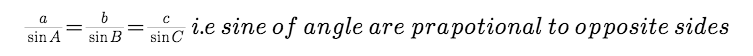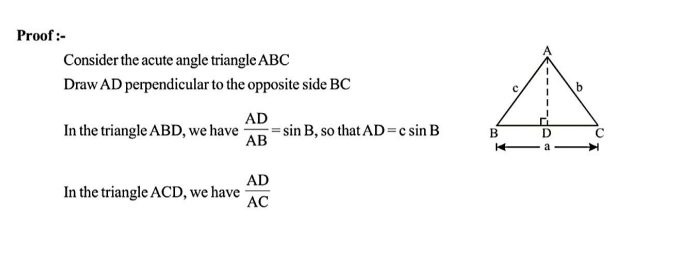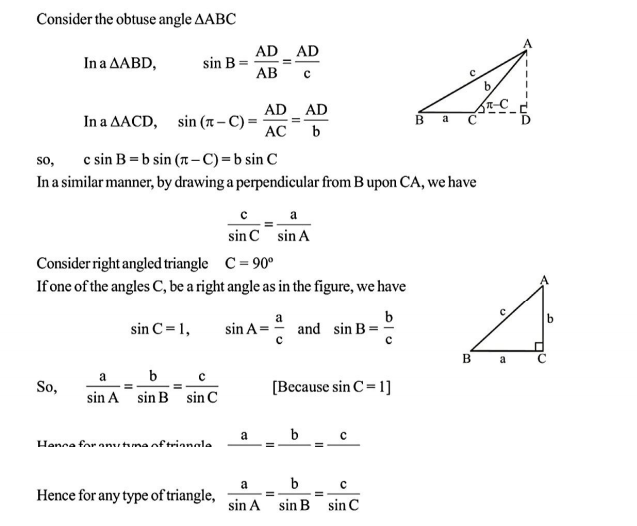Cosine Rule: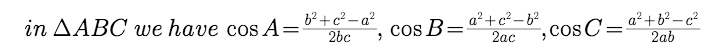where a,b,c are sides and A,B,C are angle of triangle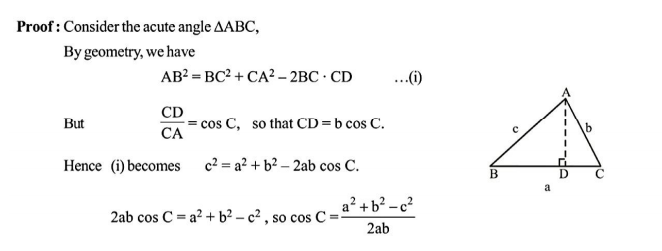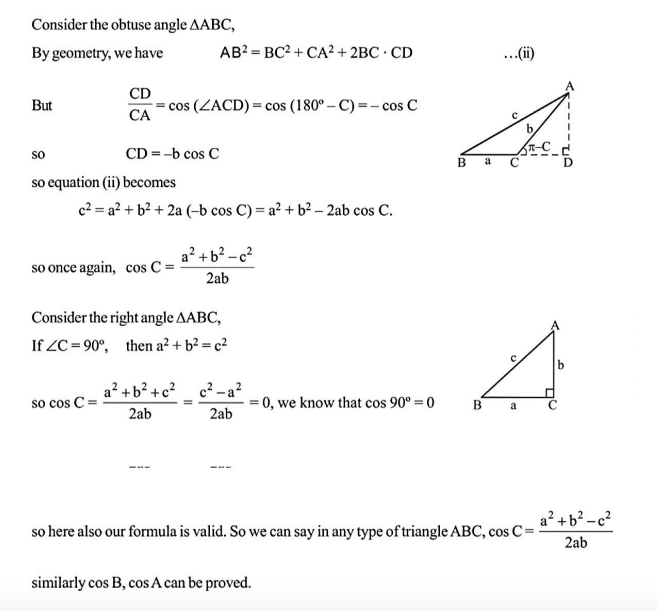MATHEMATICS SHORT TRICKS - Trigonometry Method of SubstitutionMATHEMATICS SHORT TRICKS - Arithmetic expressionMathematics Short Tricks - Quadratic Equations

No Active Challenge